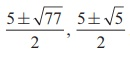Home | | Maths 12th Std | Partly Factored Polynomial

# Partly Factored Polynomial

We illustrate the method of solving this situation in the next two examples.

Partly Factored Polynomial

Quartic polynomial equations of the form (ax + b)(cx + d )( px + q)(rx + s)+ k = 0 , k ≠ 0 which can be rewritten in the form (αx2  + β x +λ) (αx2  + β x + µ)+ k = 0

We illustrate the method of solving this situation in the next two examples.

### Example 3.23

Solve the equation

(x - 2)(x - 7)(x - 3)(x + 2) +19 = 0.

### Solution

We can solve this fourth degree equation by rewriting it suitably and adopting a technique of substitution. Rewriting the equation as

(x - 2)(x - 3)(x - 7)(x + 2) +19 = 0 .

the given equation becomes

(x2 - 5x + 6)(x2 - 5x -14) +19 = 0 .

If we take x2 - 5x as y , then the equation becomes ( y + 6)( y -14) +19 = 0;

that is,

y2 - 8 y – 65 = 0 .

Solving this we get solutions y = 13 and y = -5 . Substituting this we get two quadratic equations

x2 - 5x -13 = 0 and x2 - 5x + 5 = 0

which can be solved by usual techniques. The solutions obtained for these two equations together give solutions as### Example 3.24

Solve the equation (2x - 3)(6x -1)(3x - 2)(x -12) – 7 =  0 .

### Solution

The given equation is same as

(2x - 3)(3x - 2)(6x -1)(x -12) – 7 =  0 .

After a computation, the above equation becomes

(6x2 -13x + 6)(6x2 -13x +12) – 7 =  0 .

By taking y = 6x2 -13x, the above equation becomes,

( y + 6)( y +12) - 7 = 0

which is same as

y2 +18 y + 65 = 0 .

Solving this equation, we get y = -13 and y = -5 .

Substituting the values of y in y = 6x2 -13x, we get

6x2 -13x + 5 =  0

6x2 -13x +13 =  0

Solving these two equations, we getas the roots of the given equation.

Tags : Solved Example Problems , 12th Mathematics : UNIT 3 : Theory of Equations
Study Material, Lecturing Notes, Assignment, Reference, Wiki description explanation, brief detail
12th Mathematics : UNIT 3 : Theory of Equations : Partly Factored Polynomial | Solved Example Problems